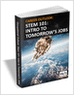MaterialsDesign CenterProcessesUnit ConversionFormulasMathematicsCalculatorsDiscussion ForumTrade PublicationsDirectory Service
CNC Machining Design Guide

Essentials of Manufacturing

Information, coverage of important developments and expert commentary in manufacturing.

STEM Career Outlook

Wages, employment opportunities, and growth projections for STEM jobs.

Learn the best principles to negotiate the salary you deserve!

more free publicationsGlossary » Units » Pressure » Kilopound Force Per Square InchKilopound Force Per Square Inch (kip/in2, ksi, KSI) is a unit in the category of Pressure. It is also known as kilopound force/square inch, kilopound per square inch. This unit is commonly used in the UK, US unit systems. Kilopound Force Per Square Inch (kip/in2, ksi, KSI) has a dimension of ML-1T-2 where M is mass, L is length, and T is time. It can be converted to the corresponding standard SI unit Pa by multiplying its value by a factor of 6894757.29.
Note that the seven base dimensions are M (Mass), L (Length), T (Time), Q (Temperature), N (Aamount of Substance), I (Electric Current), and J (Luminous Intensity).

Other units in the category of Pressure include Atmosphere (metric) (at), Atmosphere (standard) (atm), Bar (bar), Barad (barad), Barye, CentiHg (0°C), Centimeter of Mercury (0°C) (cmHg (0 °C)), Centimeter of Water (4°C) (cmH2O), Dyne Per Square Centimeter (dyn/cm2), Foot of Water (4°C) (ft H2O), Gigapascal (GPa), Hectopascal (hPa), Inch of Mercury (0°C) (inHg (0 °C)), Inch of Mercury (15.56°C) (inHg (15.56 °C)), Inch of Water (15.56°C) (inH2O (15.56 °C)), Inch of Water (4°C) (inH2O (4 °C)), Kilogram Force Per Square Centimeter (kgf/cm2), Kilogram Force Per Square Decimeter (kgf/dm2), Kilogram Force Per Square Meter (kgf/m2), Kilogram Force Per Square Millimeter (kgf/mm2), Kilopascal (kPa), Megapascal (MPa), Meter of Water (15.56°C) (mH2O, mCE (15.56 °C)), Meter of Water (4°C) (mH2O, mCE (4 °C)), Microbar (barye, Barrie) (µbar), Micron of Mercury (millitorr) (µHg (0 °C)), Millibar (mbar), Millimeter of Mercury (0°C) (mmHg, torr, Torr (0 °C)), Millimeter of Water (15.56°C) (mmH2O, mmCE (15.56 °C)), Millimeter of Water (4°C) (mmH2O, mmCE (4 °C)), Millitorr (mtorr), Newton Per Square Meter (N/m2), Ounce Force (av.) Per Square Inch (ozf/in2, osi), Pascal (Pa, N/m2), Pound Force Per Square Foot (lbf/ft2), Pound Force Per Square Inch (psi, PSI, lbf/in2), Poundal Per Square Foot (pdl/ft2), Poundal Per Square Inch (pdl/in2), Standard Atmosphere (atm), Ton Force (long) Per Square Foot (tonf/ft2 (UK)), Ton Force (long) Per Square Inch (tonf/in2 (UK)), Ton Force (metric) Per Square Centimeter (tonf/cm2 (metric)), Ton Force (metric) Per Square Meter (tonf/m2 (metric)), Ton Force (short) Per Square Foot (tonf/ft2 (US)), Ton Force (short) Per Square Inch (tonf/in2 (US)), and Torr (torr).N/ARelated PagesGlossaryCNC Machining Design GuideEssentials of Manufacturing

Information, coverage of important developments and expert commentary in manufacturing.STEM Career Outlook

Wages, employment opportunities, and growth projections for STEM jobs.